### 一、机器学习/深度学习

#### 1.1 SVM

##### 1.1.1 简述

SVM是一种二分类模型，它的基本模型是定义在特征空间上的间隔最大线性分类器，其学习策略是间隔最大化

• 硬间隔: 当训练数据线性可分时，通过硬间隔最大化可以学习得到一个线性分类器，即硬间隔SVM
• 软间隔: 当训练数据不能线性可分但是可以近似线性可分时，通过软间隔最大化也可以学习到一个线性分类器，即软间隔SVM
• 核技巧: 当训练数据线性不可分时，通过使用核技巧和软间隔最大化，可以学习到一个非线性SVM

#### 1.2 Logistic Regression (LR)

##### 1.2.2 为什么LR应用sigmoid函数?

\begin{align} p(y;\phi)&=\phi^y(1-\phi)^{(1-y)}\\ &= e^{[y \cdot log^\phi+(1-y) \cdot log(1-\phi)]} \\ & = e^{y \cdot log\frac{\phi}{1-\phi}+log(1-\phi)} \end{align}

Sigmoid优缺点：

• 优点：数据规约在[0,1]之间，有概率意义；导数易于求导；
• 缺点：$$x$$ 较大时容易导致梯度消失；本质上是一个线性分类器，处理不好特征之间相关的情况；当特征空间很大时，LR性能不是很好；
##### 1.2.3 LR模型推导

1. $$p(y|x;\theta)$$ 服从指数族分布；
2. 给了 $$x$$ ，我们的目的是为了预测 $$T(y)$$ 在条件 $$x$$ 下的期望，即 $$h(x)=E(T(y))$$
3. 参数 $$\eta$$ 和输入 $$x$$ 是线性相关的：$$\eta=\theta^Tx$$

$h(x)=E(T(y)) = E(y)$

##### 1.2.5 LR反向传播

sigmoid函数求导： $\frac{\partial \phi}{\partial \eta} = \phi(1-\phi)$$$z=p(y=1|x_i)$$，取 $$log$$ 底数为 $$e$$，则 $$\theta^*=\mathop{argmin}\limits_{\theta}\sum_{i=1}^{m}-y_ilnz_i-(1-y_i)ln(1-z_i)$$ \begin{align} \frac{\partial}{\partial \theta}(-ylnz)&=-y*\frac{1}{z}*\frac{\partial z}{\partial y}*\frac{\partial y}{\partial \theta}\\ &= -y*\frac{1}{z}*z(1-z)*x \\ & = y*(z-1)*x \end{align}

\begin{align} \frac{\partial}{\partial \theta}(-(1-y)ln(1-z))&=-1*(1-y)*\frac{1}{1-z}*(-1)*\frac{\partial z}{\partial y}*\frac{\partial y}{\partial \theta}\\ &= (1-y)*\frac{1}{1-z}*z(1-z)*x \\ & = (1-y)*z*x \end{align}

\begin{align} \frac{\partial L}{\partial \theta}&=\frac{\partial }{\partial \theta}\sum_x[-ylnz-(1-y)ln(1-z)]\\ &=\sum_x[y*(z-1)*x+(1-y)*z*x]\\ &=\sum_x(z-y)*x \end{align}

#### 1.3 GBDT & XGBoost

##### 1.3.1 简要介绍
• GBDT是一种迭代的决策树算法，由多棵决策树组成，属于 Boosting 策略。GBDT 的每一棵树都是以之前树得到的残差来更新目标值，这样每一棵树的值加起来即为 GBDT 的预测值。但是基于残差的GBDT 容易对异常值敏感，所以回归类的损失函数一般为： 绝对损失或者 Huber 损失函数。
• XGBoost是一种Boosting集成学习方法，它的核心思想就是不断地添加树，不断地进行特征分裂来生成一棵树，每次添加一个树，其实是学习一个新函数，去拟合上次预测的残差。当训练完成得到 k 棵树，我们要预测一个样本的分数，其实就是根据这个样本的特征，在每棵树中会落到对应的一个叶子节点，每个叶子节点就对应一个分数，最后只需要将每棵树对应的分数加起来就是该样本的预测值。
##### 1.3.2 XGBoost相比GBDT的优化
• GBDT只支持决策树，XGBoost除了支持决策树，可以支持多种弱学习器；其次GBDT损失函数化简的时候进行的是一阶泰勒公式的展开，而XGBoost使用的是二阶泰勒公式的展示。还有一点是XGBoost的目标函数加上了正则项，这个正则项是对树复杂度的控制，防止过拟合。
• 从最优化的角度来看，GBDT采用的是数值优化的思维, 用的最速下降法去求解损失函数的最优解, 其中用CART决策树去拟合负梯度，用牛顿法求步长；XGboost用的解析的思维, 对损失函数展开到二阶近似, 求得解析解, 用解析解作为增益来建立决策树, 使得损失函数最优。

#### 1.4 集成学习

##### 1.4.1 定义

1. 以何种方式训练多个个体学习器；
2. 以何种方式将训练好的学习器结合起来；
##### 1.4.2 集成学习分类
• Bagging: 采用自助采样法，也就是有放回的采样。通过采样得到多个数据集，并在每个数据集上训练一个基分类器，然后将各分类器的结果结合起来得到最终预测结果（一般分类采取简单投票，回归采取简单平均）。代表算法有随机森林等。
• Stacking: 训练一个元模型用于组合各个基模型。具体来说就是将训练好的各个基模型的输出作为元模型的输入来训练一个元模型，这样就能得到一个最终的输出。
##### 1.4.3 集成学习优点
• 从统计的方面来看，由于学习任务的假设空间往往很大，可能有多个假设在训练集上达到同等性能，此时若使用单学习器可能因误选而导致泛化性能不佳，结合多个学习器则会减小这一风险；
• 从计算的方面来看，学习算法往往会陷入局部极小，有的局部极小点对应的泛化性能可能很糟糕，而通过多次运行之后进行结合，可降低陷入糟糕局部极小点的风险；
• 从表示的方面来看，某些学习任务的真实假设可能不在当前学习算法所考虑的假设空间中，此时若使用单学习器则肯定无效，而通过结合多个学习器，由于相应的假设空间有所扩大，有可能学得更好的近似。

##### 1.4.4 Bagging和Boosting比较
1. 样本选择
• Bagging: 训练集是有放回选取的，从原始集中选出的各轮训练集之间是独立的。
• Boosting: 每一轮的训练集不变，只是训练集中每个样例在分类器中的权重发生变化，而权重是根据上一轮的分类结果进行调整的。
2. 样本权重
• Bagging: 使用均匀取样，每个样例的权重相等。
• Boosting: 根据错误率不断调整样例的权值，错误率越大则权重越大。
3. 弱分类器
• Bagging: 所有弱分类器的权重相等。
• Boosting: 每个弱分类器都有相应的权重，对于分类误差小的分类器会有更大的权重。
4. 并行计算
• Bagging: 所有弱分类器可以并行生成。
• Boosting: 各个弱分类器只能顺序生成，因为后一个模型参数需要前一轮模型的结果。
5. 优化部分
• Bagging: 主要减小模型的方差。
• Boosting: 主要减小模型的偏差。
##### 1.4.5 为什么Bagging减小方差，Boosting减小偏差？
• Bagging是对许多强（甚至过强）的分类器求平均。在这里，每个单独的分类器的bias都是低的，平均之后bias依然低；而每个单独的分类器都强到可能产生overfitting的程度，也就是variance高，求平均的操作起到的作用就是降低这个variance。
• Boosting是迭代算法，每一轮迭代都根据前面迭代模型的效果来进行修正，进行样本或分类器的加权。这个过程可以理解为一步一步逼近真实值。因此如果迭代次数足够多，可以产生更好的预测结果，也就是减小了bias。

#### 1.5 过拟合和欠拟合

##### 1.5.1 避免过拟合的方法
• 增加训练数据或减少不必要的特征；
• 降低模型复杂度如Dropout或剪枝；
• 采用 Bagging 或者 Stacking 的集成方法；
• 标签平滑；
• 加入正则化项并提高正则化项系数；
• Early Stopping；
##### 1.5.2 避免欠拟合的方法
• 添加新特征；
• 增加模型复杂度如线性模型添加高次项，神经网络增加网络层数或神经元个数；
• 减少正则化项的系数；

#### 1.7 正则化

##### 1.7.1 $$L_1$$ 和 $$L_2$$ 正则化
• $$L_1$$ 正则化: $$\lambda\sum_{j=1}^n|\omega_j|$$ ; $$L_2$$ 正则化: $$\lambda\sum_{j=1}^n \omega_j^2$$
##### 1.7.2 $$L_1$$ 正则化和 $$L_2$$ 正则化对比
1. 从优化的角度

• $$L_1$$ 正则化容易产生稀疏矩阵，即参数容易是0；

• $$L_2$$ 正则化容易产生平滑矩阵，即参数尽可能的小但不是0；

2. 从先验的角度

• $$L_1$$ 正则化引入拉普拉斯分布
• $$L_2$$ 正则化引入高斯分布
##### 1.7.3 为什么 $$L_1$$ 正则化会产生稀疏解？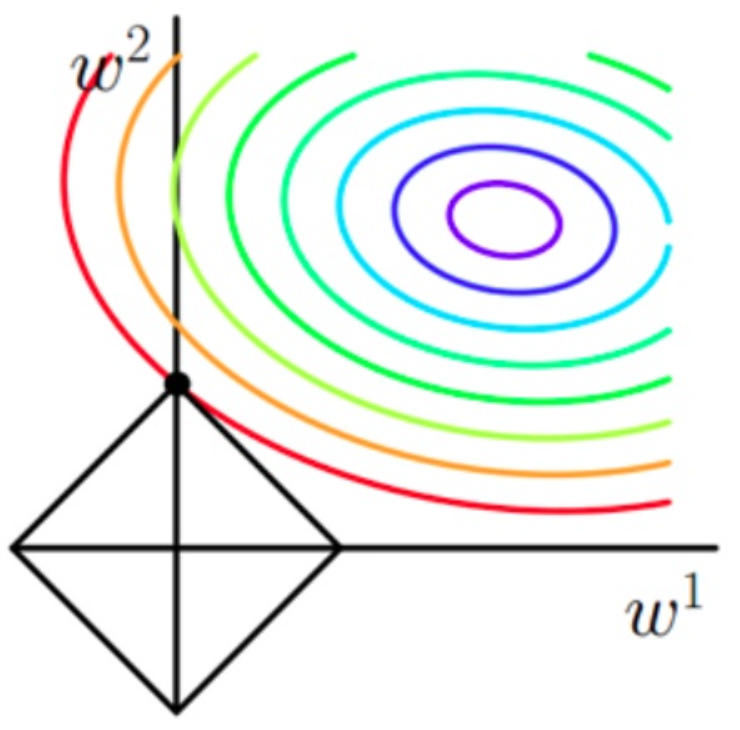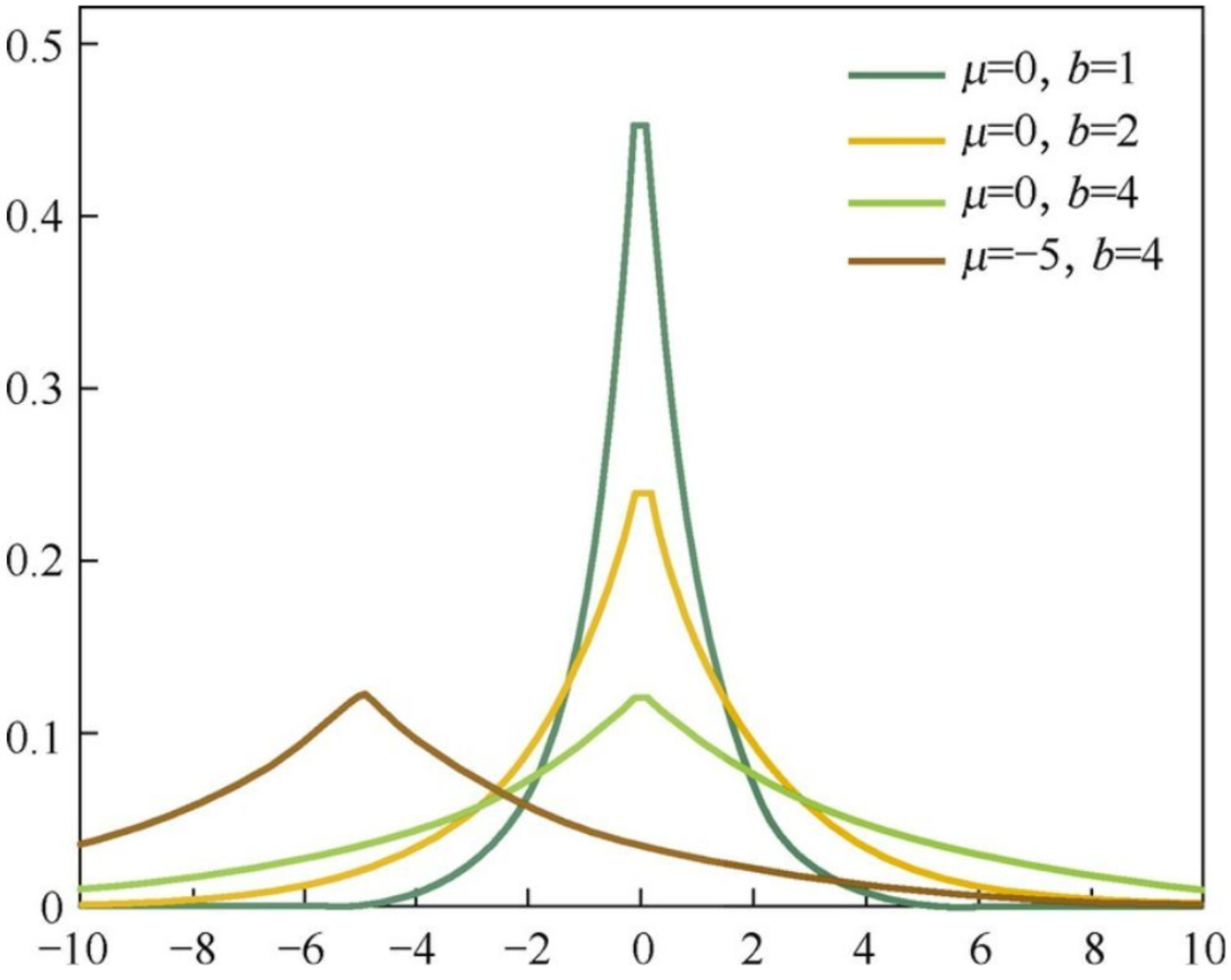##### 1.7.4 为什么 $$L_2$$ 正则化会避免过拟合？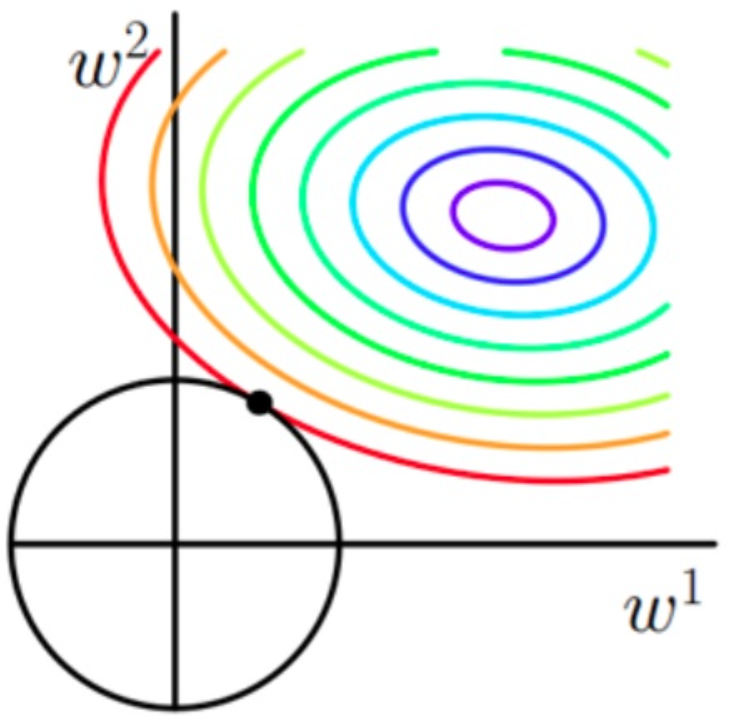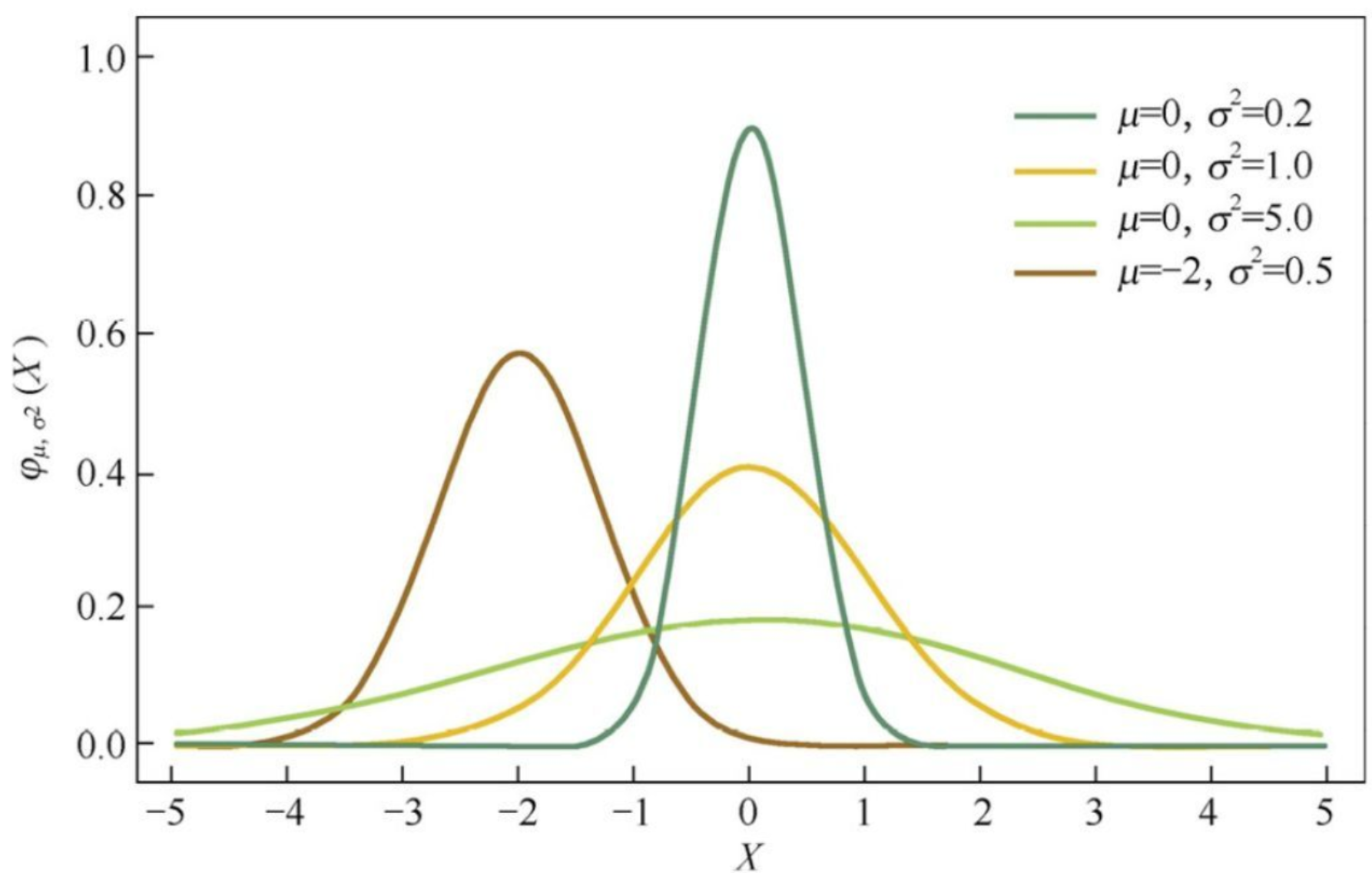#### 1.8 初始化

##### 1.8.1 初始化方法有哪些？
• 随机初始化: 随机参数服从高斯分布或均匀分布，即 $$\omega\sim N(0,1)$$$$\omega\sim U$$

• Xavier初始化：激活值的方差是逐层递减的，这导致反向传播中的梯度也逐层递减。要解决梯度消失，就要避免激活值方差的衰减，最理想的情况是，每层的输出值（激活值）保持高斯分布，即 $\omega \sim N(0, \frac{1}{\sqrt{n}}), n=\frac{n_{in}+n_{out}}{2}$ 如果是均匀分布，则参数的随机取值范围为:

$\omega \sim U[-\sqrt{\frac{6}{n_{in}+n_{out}}}, \sqrt{\frac{6}{n_{in}+n_{out}}}]$

• He初始化: 当使用ReLU作为激活函数时，Xavier的效果并不好。原因在于，当ReLU的输入小于 0 时，其输出为 0，相当于该神经元被关闭了，影响了输出的分布模式。因此He初始化在Xavier的基础上，假设每层网络有一半的神经元被关闭，于是其分布的方差也会变小。经过验证发现当对初始化值缩小一半时效果最好。 $\omega \sim N(0,\sqrt{\frac{2}{n_i}})$

#### 1.9 激活函数

##### 1.9.1 Sigmoid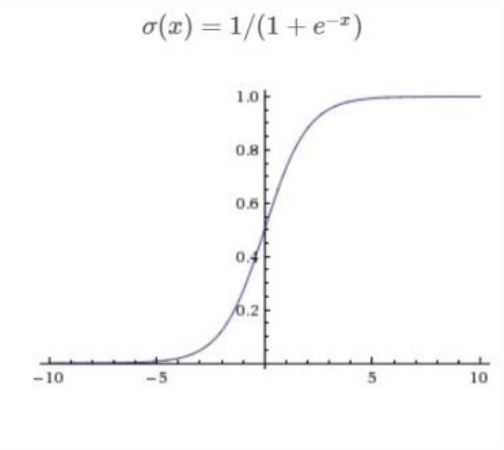• $$\sigma'(x)=\sigma(x)(1-\sigma(x))\in[0,0.25]$$
• 能够把输入的连续实值变换为0到1之间的输出；

• 当神经元的激活在接近 0 或 1 处时会饱和，在这些区域梯度几乎为0，这就会导致梯度消失；
• 解析式中含有幂运算，求解时相对来讲比较耗时；
• Sigmoid的输出不是零中心的，关于参数 $$\omega$$ 各分量在梯度更新的过程中，将会同时增大或减小，如果随机初始化到第四象限，将会导致权重更新时出现低效率 z 字型梯度更新趋势；
##### 1.9.2 Tanh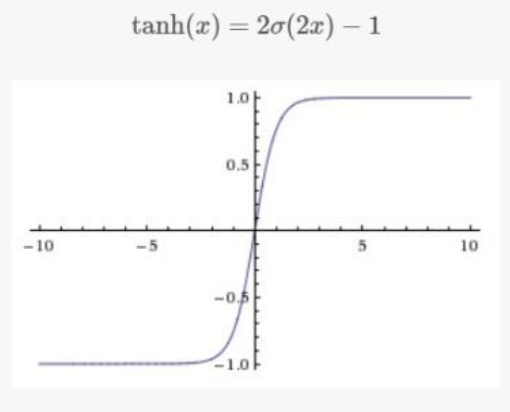• 相比Sigmoid函数收敛速度更快；
• 相比Sigmoid函数，其输出以 0 为中心，即 (-1,1) 而不是 (0,1) ；

• 还是存在饱和性梯度消失的问题；
##### 1.9.3 ReLU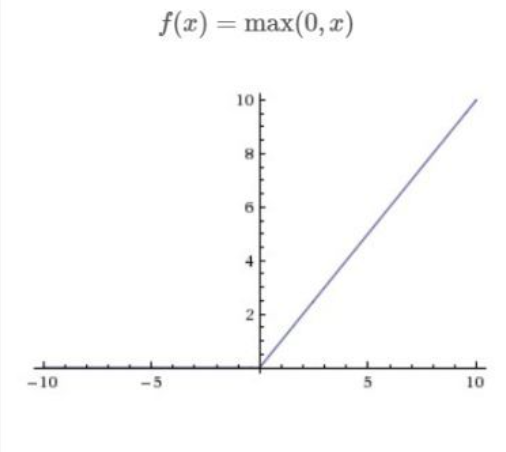• 可以有效缓解梯度消失或爆炸的问题；
• 计算效率高，因为Sigmoid和Tanh中包含指数运算；
• 收敛速度快于Sigmoid和Tanh；

• 由于负数部分恒为 0，会导致一些神经元无法激活（可通过设置小学习率部分解决）；
• 输出不是以 0为中心的；
##### 1.9.4 Leaky ReLU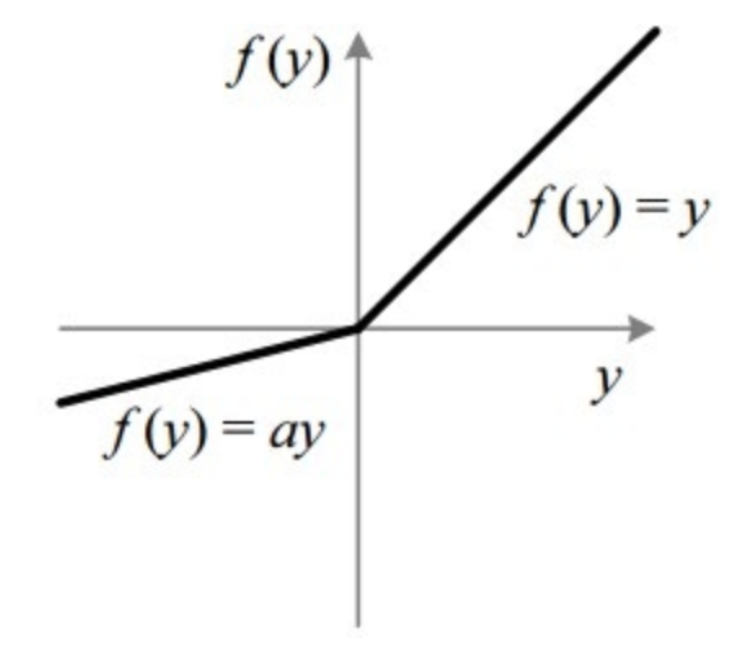• 解决了ReLU的神经元死亡问题，在负半轴具有小的正斜率，因此对于负输入值，它也可以进行反向传播；
• 可以有效缓解梯度消失或爆炸的问题；
• 计算效率高，因为Sigmoid和Tanh中包含指数运算；
• 收敛速度快于Sigmoid和Tanh；

• 在不同区间应用的不同函数所带来的不一致结果，无法为正负输入值提供一致的关系预测；
• 大量的实践证明，其效果不稳定，故实际中该函数的应用并不多；

#### 1.10 损失函数

##### 1.10.1 常见的损失函数

（1）用于回归的损失函数:

• 平均绝对误差MAE: a. 对于离群点不过分敏感，拟合直线能够较好地表征正常数据的分布情况；b. 训练时梯度始终很大且在零点处连续但不可导；c. 模型学习速度慢，训练结束时可能会遗漏全局最小值。
• 均方误差MSE: a. 随着误差的减小，梯度也在减小，收敛速度快；b. 无法避免离群点可能导致的梯度爆炸问题；

（2）用于分类的损失函数:

• 0-1损失zero-one: 如果预测值与目标值不相等，那么为1，否则为0。该损失函数不考虑预测值和真实值的误差程度，也就是只要预测错误，预测错误差一点和差很多是一样的。可以看出上述的定义太过严格，如果真实值为1，预测值为0.999，那么预测应该正确，但是上述定义显然是判定为预测错误。

• 指数损失: $$L(y_i,\hat{y}_i)=e^{-y_i\cdot \hat{y}_i},y_i\in\{-1,1\}$$。对离群点、噪声非常敏感，常用于Boosting算法中，如AdaBoost。

• 合页损失Hinge: $$L(y_i,\hat{y}_i)=max(0,1-y_i\cdot \hat{y}_i),y_i\in\{-1,1\}$$。用来解决间隔最大化问题，如在SVM中解决几何间隔最大化问题。

• 交叉熵损失函数: a. 本质上是一种对数似然函数，可用于二分类或多分类任务中；b. 当使用Sigmoid作为激活函数的时候，常用交叉熵损失函数而不用均方误差损失函数，因为它可以完美解决平方损失函数权重更新过慢的问题，具有“误差大的时候，权重更新快；误差小的时候，权重更新慢”的良好性质；

##### 1.10.2 交叉熵函数和最大似然函数的联系和区别？
• 区别: 交叉熵函数使用来描述模型预测值和真实值的差距大小，越大代表越不相近；似然函数的本质就是衡量在某个参数下，整体的估计和真实的情况一样的概率，越大代表越相近。

• 联系: 交叉熵函数可以由最大似然函数在伯努利分布的条件下推导出来，或者说最小化交叉熵函数的本质就是对数似然函数的最大化

##### 1.10.3 用Sigmoid作为激活函数的时候，为什么要用交叉熵损失而不用均方误差损失？

$\frac{\partial C}{\partial b}=(a-y)\sigma'(z)$

$b=b-\eta\frac{\partial C}{\partial b}=b-\eta(a-y)\sigma'(z)$

$b=b-\eta\frac{\partial C}{\partial b}=b-\eta\frac{\partial C}{\partial z}\frac{\partial z}{\partial b}=b-\eta (a-y)$

##### 1.10.4 交叉熵损失函数和均方损失函数的区别？
1. 概念不一样

均方损失是求 $$n$$ 个样本的 $$n$$ 个输出与期望输出的差的平方的平均值；交叉熵损失描述模型预测值和真实值的差距大小，越大代表越不相近。

2. 参数更新不一样

Sigmoid作为激活函数时，均方误差损失梯度更新缓慢；而交叉熵损失的参数更新只和误差有关，误差大的时候权重更新快，误差小的时候权重更新慢。

3. 使用场景不一样

MSE更适合回归问题，交叉熵更适合分类问题。

4. Sigmoid/Softmax作为激活函数时，如果采用均方误差损失函数，那么这是一个非凸优化问题，不宜求解；而采用交叉熵损失函数则是一个凸优化问题，更容易优化求解。

##### 1.10.6 交叉熵的设计思想是什么？
• 交叉熵被用来描述模型预测值和真实值的差距大小，越大代表越不相近。其本质是对数函数，相当于在最大似然估计前加了一个负号，最大化似然估计就是最小化交叉熵损失函数；

• 交叉熵损失函数可以完美解决均方损失函数权重更新过慢的问题，具有“误差大的时候，权重更新快；误差小的时候，权重更新慢”的良好性质；

• 交叉熵损失函数在逻辑回归和多分类任务上被广泛使用；

##### 1.10.8 为什么分类问题不能使用MSE作为损失函数？
1. 从损失函数的物理意义来说
• MSE衡量的是预测值和目标值的欧式距离
• 交叉熵是一个信息论的概念，能够衡量同一个随机变量中的两个不同概率分布的差异程度。在机器学习中表示为真实概率分布与预测概率分布之间的差异，交叉熵的值越小，模型预测效果就越好；
• 分类问题中 label 的值大小在欧氏空间中是没有意义的，所以分类问题不能用 MSE 作为损失函数；
2. 从优化求解角度来说
• 分类问题通常使用 Sigmoid 作为激活函数，这样 MSE 存在的连乘项有可能会导致梯度消失
• MSE 函数对于二分类问题来说是非凸的，不宜求解；
3. 从数据分布角度来说
• 如果使用 MSE 作为损失函数，意味着假设数据采样误差是遵循正态分布的，即做了高斯先验的假设；
• 如果假设误差遵循正态分布，并使用最大似然估计，我们将会得出 MSE 就是优化模型的损失函数；
• 但是实际上二分类的数据集遵循伯努利分布

#### 1.11 信息论

##### 1.11.1 熵
1. 信息熵

用来度量信息的不确定程度。信息量越大，熵越大；不确定程度越低，熵越小。

$H(X)=-\sum_{i=1}^{n}p(x_i)logp(x_i)$

1. 条件熵

在一个条件下，随机变量的不确定性。

\begin{align} H(X|Y) &= \sum_{x\in X}p(x)H(Y|X=x)\\ &=-\sum_{x\in X}p(x)\sum_{y\in Y} p(y|x)logp(y|x)\\ &=-\sum_{x\in X}\sum_{y\in Y}p(x,y)logp(x|y) \end{align}

2. 互信息 (信息增益)

两个随机变量之间的相关程度。条件熵越大，互信息越小，条件熵越小，互信息越大。

$I(X;Y)=H(X)-H(X|Y)$

3. 联合熵

两个随机变量一起发生时产生的信息量。

$H(X,Y)=-\sum_{x,y}p(x,y)log(x,y)=H(X|Y)+H(Y)$

4. 相对熵 (KL散度)

衡量两个概率分布之间的差异

$D_{KL}(p||q)=\sum_{x}p(x)log(\frac{p(x)}{q(x)})$

##### 1.11.2 交叉熵和相对熵的区别

\begin{align} D_{KL}(p||q)&=\sum_{i=1}^{n}p(x_i)log(\frac{p(x_i)}{q(x_i)})\\ &=\sum_{i=1}^{n}p(x_i)log(p(x_i))-\sum_{i=1}^{n}p(x_i)log(q(x_i)) \\ &=-H(X)+[-\sum_{i=1}^{n}p(x_i)log(q(x_i))]\\ &= [-\sum_{i=1}^{n}p(x_i)log(q(x_i))]-H(X) \end{align}

#### 1.12 归一化

##### 1.12.1 归一化的方法有哪些？
• Min-Max 归一化 $X = \frac{X_i-X_{min}}{X_{max}-X_{min}}\in[0,1]$

将特征映射到0和1之间，适用在数值比较集中的情况，但该方法对异常值（极值）比较敏感。

• Z-Score标准化 $X = \frac{X-\mu}{\sigma}$

将特征缩放为均值为0，方差为1的标准正态分布。

• 非线性归一化

适用在数据分化比较大的场景，通过一些数学函数，将原始值进行映射，如log，exp，tan等。

##### 1.12.2 Min-Max归一化和Z-Score标准化的联系和区别？
• 联系

本质上都是对数据的线性变换；

• 区别

1. Min-Max归一化映射后的数据范围集中在[0,1]区间内，而Z-Score标准化变换后的数据没有范围；

2. Min-Max归一化的缩放比例仅仅和极值有关，而Z-Score标准化和每个点都有关系，通过均值和方差体现出来。

##### 1.12.3 为什么要做归一化？
• 统计建模中，如回归模型，特征的量纲不一致导致了回归系数无法解读或错误解读，因此需要将它们变换到同一量纲下；
• 机器学习任务中有很多地方涉及到距离的计算，如PCA、KNN、KMeans等等。不同维度量纲不同可能导致距离的计算依赖于量纲较大的那些特征而得到不合理的结果；
• 在使用梯度下降的方法求解最优化问题时，归一化/标准化后可以加快梯度下降的求解速度，即提升模型的收敛速度。
##### 1.12.4 哪些算法需要归一化？
• 需要归一化: 需要使用梯度下降和计算距离的模型要做归一化，因为不做归一化会使收敛的路径程 z 字型下降，导致收敛速度太慢，且不容易找到最优解。归一化之后加快了梯度下降求最优解的速度，并有可能提高精度。比如说线性回归、逻辑回归、KNN、KMeans、神经网络等最优化问题。一般算法如果本身（计算距离）受量纲影响较大，或者相关优化函数受量纲影响大，则需要进行特征归一化。

• 不需要归一化: 概率模型、树形结构模型不需要归一化，因为它们不关心变量的值，而是关心变量的分布和变量之间的条件概率，如决策树、随机森林。

#### 1.13 梯度下降

每一次迭代使用所有样本来进行梯度的更新

优点

• 一次迭代是对所有样本进行计算，此时利用矩阵进行操作，实现了并行；
• 由全数据集确定的方向能够更好地代表样本总体，从而更准确地朝向极值所在的方向。当目标函数为凸函数时，BGD一定能够得到全局最优；

缺点

• 当样本数目很大时，每迭代一步都需要对所有样本计算，训练过程会很慢；
• 从迭代的次数上来看，BGD迭代的次数相对较少；

每次迭代使用一个样本来对参数进行更新

优点

• 由于不是在全部训练数据上实现的梯度下降，而是在每轮迭代中，随机优化某一条训练数据，这样每一轮参数的更新速度大大加快；

缺点

• 准确度下降。即使在目标函数为强凸函数的情况下，SGD仍旧无法做到线性收敛；
• 可能会收敛到局部最优，因为单个样本并不能代表全体样本的趋势；
• 不易于并行实现；

每次迭代使用batchsize个样本来对参数进行更新

优点

• 通过矩阵运算，每次在一个batch上优化神经网络参数并不会比单个数据慢太多；
• 每次使用一个batch可以大大减小收敛所需要的迭代次数，同时可以使收敛到的结果更加接近梯度下降的效果；
• 可实现并行化；

缺点

• batchsize的不当选择可能会带来一些问题；

#### 1.14 梯度消失和梯度爆炸

##### 1.14.2 梯度消失和梯度爆炸的影响
• 梯度消失: a. 当梯度消失发生时，接近于输出层的隐藏层由于其梯度相对正常，所以权值更新时也就相对正常；b. 但是当越靠近输入层时，由于梯度消失现象，会导致靠近输入层的隐藏层权值更新缓慢或者更新停滞
• 梯度爆炸: a. 当梯度爆炸发生时，初始的权值过大，靠近输入层的权值变化比靠近输出层的权值变化更快，就会引起梯度爆炸的问题；b. 会导致模型不稳定，更新过程中的损失出现显著变化；c. 也可能导致训练过程中，模型损失变成 NaN；
##### 1.14.3 梯度消失的解决方案
• 换成ReLU激活函数：Sigmoid 作为激活函数更容易出现梯度消失的问题，为了缓解这样的问题可以使用 ReLU 及其变形作为激活函数。ReLU激活函数的导数为1，那么就不存在梯度消失或爆炸的问题了，每层的网络都可以得到相同的更新速度；
• 残差结构：因为残差的存在，梯度可以传播的“更远”，靠近输入的部分网络结构也可以通过梯度下降更新网络；
• 门控网络：在RNN中，每个记忆单元都会乘上一个参数矩阵和激活函数的导数，这种连乘使得记忆衰减的很快，而LSTM通过记忆和当前输入”相加”，使得之前的记忆会继续存在而不是受到乘法的影响而部分“消失”，因此不会衰减；
##### 1.14.4 梯度爆炸的解决方案
• 梯度截断：设置一个梯度截断阈值，当更新梯度的时候，如果梯度超过了这个阈值，那么就将其强制限制在这个范围之内；
• 换成ReLU激活函数：激活函数的导数为1，那么久不存在梯度消失或爆炸的问题了，每层的网络都可以得到相同的更新速度；
• 权重正则化：如果发生梯度爆炸，那么权重的范数就会变的非常大，反过来，通过限制正则化项的大小，也可以在一定程度上限制梯度爆炸的发生；

#### 1.15 优化器

##### 1.15.1 说说常见的优化器及优化思路？
1. 梯度下降法

梯度下降的核心思想：负梯度方向是使函数值下降最快的方向，因此我们的目标就是求取目标函数的负梯度。 在梯度下降法中，因为每次都遍历了完整的训练集，其能保证结果为全局最优，但是也因为我们需要对于每个参数求偏导，且在对每个参数求偏导的过程中还需要对训练集遍历一次，当训练集很大时，计算费时。 $w_j^* = w_j + \eta \cdot \nabla w_j$

2. 批量梯度下降法

为了解决梯度下降法的耗时问题，批量梯度下降法在计算梯度时，不用遍历整个训练集，而是针对一个批次的数据。

3. 随机梯度下降法(SGD)

每次从训练集中随机抽取一个样本来计算梯度。因此，其速度较快，但是其每次的优化方向不一定是全局最优的。所以每一次迭代的梯度受抽样的影响比较大，也就是说梯度含有比较大的噪声，不能很好的反映真实梯度，并且SGD无法逃离鞍点。

4. Momentum随机梯度下降法(MSGD)

Momentum借用了物理中的动量概念，即前一次的梯度也会参与运算。为了表示动量，引入了一阶动量 $$m$$(momentum)，$$m$$ 是之前的梯度的累加,但是每回合都有一定的衰减 。总而言之，momentum能够加速SGD收敛，抑制震荡，并且动量有机会逃脱局部极小值(鞍点)。 $m_t = \beta\cdot m_{t-1}+(1-\beta)\cdot g_t$

$w_{t+1} = w_t-\eta\cdot m_t$

注：$$\beta$$ 表示动量因子，一般取 0.9，$$g_t$$ 表示第 $$t$$ 次 epoch 计算的梯度。

SGD 的学习率是线性更新的，每次更新的差值一样，后面的优化法开始围绕自适应学习率进行改进。 AdaGrad 法引入二阶动量，根据训练轮数的不同，对学习率进行了动态调整。但缺点是 AdaGrad 法仍然需要人为指定一个合适的全局学习率，同时网络训练到一定轮次后，分母上梯度累加过大使得学习率为 0 而导致训练提前结束。 $v_t = \sqrt{\sum_{\tau=1}^tg_{\tau}^2+\epsilon}$

$\eta_t = \frac{\eta_{global}}{v_t}$

$w_{t+1}=w_t-\eta_t \cdot g_t$

注：$$g_t$$ 表示第 $$t$$ 次 epoch 计算的梯度，$$\epsilon$$ 为一个小常数（通常设定为1e-6数量级）以防止分母为 0 。在网络训练的前期，由于分母中梯度的累加 $$v_t$$ 较小，所以一开始的学习率 $$\eta_t$$ 比较大；随着训练后期梯度累加较大时， $$\eta_t$$ 逐渐自适应的减小。

AdaDelta 法是对 AdaGrad 法的扩展，通过引入衰减因子 $$\rho$$ 消除 AdaGrad 法对全局学习率的依赖，而且只用了部分梯度加和而不是所有，这样避免了梯度累加过大使得学习率为 0 而导致训练提前结束。 $v_t = \rho \cdot v_{t-1} + (1-\rho) \cdot g_t^2$

$\eta_t = \frac{\sqrt{s_{t-1}+\epsilon}}{\sqrt{v_t+\epsilon}}$

$s_t = \rho \cdot s_{t-1}+(1-\rho)\cdot(\eta_t\cdot g_t)^2$

注：$$\rho$$ 为区间 [0,1] 的实值，较大的 $$\rho$$ 会促进网络更新，较小的 $$\rho$$ 会抑制网络更新。两个超参数的推荐设定为 $$\rho=0.95,\epsilon=10^{-6}$$

7. RMSProp

AdaGrad 算法在迭代后期由于学习率过小，可能较难找到一个有用的解。为了解决这一问题，RMSProp 算法对 AdaGrad 算法做了一点小小的修改，RMSProp 使用指数衰减只保留过去给定窗口大小的梯度，使其能够在找到凸状结构后快速收敛。RMSProp法可以视为 AdaDelta 法的一个特例，其缺陷在于依然使用了全局学习率，需要根据实际情况来设定。可以看出分母不再是一味的增加，它会重点考虑距离它较近的梯度（指数衰减的效果）。优点是只用了部分梯度加和而不是所有，这样避免了梯度累加过大使得学习率为 0 而导致训练提前结束。 $v_t = \rho \cdot v_{t-1} + (1-\rho) \cdot g_t^2$

$\eta_t = \frac{\eta_{global}}{\sqrt{v_t+\epsilon}}$

$w_{t+1}=w_t-\eta_t\cdot g_t$

注：实际使用中推荐参数设定为 $$\eta_{global}=1,\rho=0.9,\epsilon=10^{-6}$$

Adam 法本质上是带有动量项的RMSProp法，它利用梯度的一阶矩估计和二阶矩估计动态调整每个参数的学习率。Adam 法主要的优点在于经过偏置校正后，每一次迭代学习率都有一个确定范围，这样可以使得参数更新比较平稳$m_t=\beta_1\cdot m_{t-1}+(1-\beta_1)\cdot g_t$

$v_t=\beta_2\cdot v_{t-1}+(1-\beta_2)\cdot g_t^2$

$m_t^*=\frac{m_t}{1-\beta_1^t}$

$v_t^*=\frac{v_t}{1-\beta_2^t}$

$w_{t+1}=w_t-\eta\frac{m_t^*}{\sqrt{v_t^*}+\epsilon}$

注：实际使用中推荐参数设定为：$$\beta_1=0.9,\beta_2=0.999,\epsilon=10^{-8},\eta=0.001$$

##### 1.15.3 Batch的大小如何选择，过大的batch和过小的batch分别有什么影响？
1. 在合理范围内，增大 batchsize 的好处：
• 提高了内存利用率以及大矩阵乘法的并行化效率；
• 减少了跑完一次 epoch 所需要的迭代次数，加快了对于相同数据量的处理速度；
2. 盲目增大 batchsize 的坏处：
• 提高了内存利用率，但是内存容量可能不足。
• 跑完一次 epoch 所需的迭代次数减少，要想达到相同的精度，其所花费的时间大大增加，从而对参数的修正也就显得更加缓慢；
• batchsize 增大到一定程度，其确定的下降方向已经基本不再变化；
3. batchsize 过小的影响：
• 训练时不稳定，可能不收敛；
• 精度可能更高；

#### 1.16 显存爆炸

• 模型参数：模型越大，所占用显寸越多。
• 缓存变量：默认情况下，会保存模型每一层的中间计算结果。比如ReLU如果没有指定 inplace=True 那么就会产生中间计算结果，这些中间结果是用于梯度反向传播的时候用到的，没有这些缓存就计算不了梯度。

• 减少 batchsize

• 使用 DataParallel 数据并行（即多显卡训练）；

• validation时 with torch.no_grad()

• 在不带来错误的情况下多用 inplace=True

• 梯度累积：每次获取1个batch的数据，计算1次梯度，梯度不清空，不断累加，累加一定次数后，根据累加的梯度更新网络参数，然后清空梯度，进行下一次循环；

• 重计算：重计算本质上是一种用时间换空间的策略，可以将它类比成一种 Tensor 缓存策略， 当显存空间不足时，可以选择把一些前向计算的结果清除。当需要再次用到这些计算结果时，再根据之前缓存的检查点（Checkpoint）去重新计算它们；• 混合精度训练；

#### 1.17 学习率

##### 1.17.1 学习率衰减

1. 等步长衰减

每训练 step_size 个 epoch，学习率调整为 $$lr=lr*\gamma$$.

2. 多步长衰减

跟等步长衰减类似，但学习率调整的间隔并不是相等的，如 epoch 为10时调整一次，30时调整一次，80时调整一次…

3. 指数衰减

学习率呈指数型衰减，每训练一个epoch，$$lr=lr*\gamma^{epoch}$$.

4. 余弦衰减

学习率呈余弦函数型衰减。

##### 1.17.2 Warmup学习率预热

Warmup是针对学习率优化的一种策略，主要过程是，在训练开始的时候先选择使用一个较小的学习率训练一些epoch，在预热期间学习率逐步增加至预先设置的lr ，之后的训练过程中再使lr逐步降低。

• Warmup的作用？

由于刚开始训练时,模型的权重是随机初始化的，此时若选择一个较大的学习率，可能带来模型的不稳定(振荡)，选择Warmup预热学习率的方式，可以使得开始训练的几个epoch学习率较小，在预热的小学习率下，模型可以慢慢趋于稳定，等模型相对稳定后再选择预先设置的学习率进行训练，使得模型收敛速度变得更快，模型效果更佳。

• 为什么Warmup有效？

目前还没有很严谨的理论证明，只有一些直觉上的解释。

在网络训练前期，初始化的权重离最优权重距离较远，因此loss很大，计算出来的梯度也很大，此时如果使用较大的学习率，反而会让网络的优化过犹不及，距离最优点越来越远，通俗来说就是“跑飞了”。有些研究表明，训练初期跑飞了，后期不一定能拉的回来。因此在训练初期，loss很大（或者说梯度很大）的时候，更适合使用较小的学习率，等到网络学会了一些知识，loss不那么大了，就可以换用更大的学习率，然后按照正常规则慢慢衰减。

#### 1.18 样本不均衡

1. 重新采样训练集

• 欠采样：通过减少多数类的大小来平衡数据集。缺点是会丢失多数类的一些重要信息，不能够充分利用已有的信息。
• 过采样：增加少数类样本，最简单的办法是简单复制少数类样本，缺点是可能导致过拟合，没有给少数类增加任何新的信息。改进的方法有通过在少数类中加入随机高斯噪声或产生新的合成样本等方法。
2. 带权重的Loss

• 在损失函数中增大对稀有类别分类错误的惩罚权重（如Focal Loss） $FL(p_t)=-\alpha_t(1-p_t)^\gamma log(p_t)$

注：$$\alpha$$ 控制正负样本的权重，$$(1-p_t)^\gamma$$ 控制容易分类和难分类样本的权重。

#### 1.19 数据预处理

##### 1.19.1 缺失值
• 不处理（缺失比例较小且选择的模型能够自动处理缺失数据，如XGBoost）；

• 删除整行或整列的数据；

• 利用其他值去填充这些缺失值：

• 均值插补：如果样本属性的距离是可度量的，则使用该属性有效值的平均值来插补缺失的值；如果距离是不可度量的，则使用该属性有效值的众数来插补缺失的值。
• 同类均值插补：首先将样本进行分类，然后以该类中样本的均值来插补缺失值。
• 建模预测：将缺失的属性作为预测目标来预测，将数据集按照是否含有特定属性的缺失值分为两类，利用现有的机器学习算法对待预测数据集的缺失值进行预测（该方法的根本缺陷是如果其他属性和缺失属性无关，则预测的结果毫无意义；但是若预测结果相当准确，则说明这个缺失属性是没必要纳入数据集中的）；
##### 1.19.2 异常值

• 偏差检测，如聚类、最近邻等；
• 基于统计的异常点检测算法，如极差、四分位数间距、均差、标准差等；
• 基于距离的异常点检测算法，主要通过距离方法来检测异常点，将数据集中与大多数点之间距离大于某个阈值的点视为异常点，主要使用的距离度量方法有绝对距离 ( 曼哈顿距离 ) 、欧氏距离和马氏距离等方法；
• 基于密度的异常点检测算法，考察当前点周围密度，可以发现局部异常点，例如LOF算法；
##### 1.19.3 类别型特征
• 序号编码：一般用来处理类别间具有大小关系的数据；
• 独热编码：使用稀疏向量节省空间（如{10,[4,6],[1,3]}代表{长度,非零下标,零值下标}），配合特征选择降低维度（a. 高维度特征对距离很难得到有效衡量，b. 参数量随着维度增高而增加，容易引发过拟合，c. 只有部分维度对分类、预测有帮助）；
• 二进制编码：本质是利用二进制对ID进行哈希映射，其维数小于独热编码，节省存储空间；
##### 1.19.4 如何将数值型特征转为类别型特征（特征离散化）？
1. 离散特征的增加和减少都较容易，有利于模型迭代；
2. 降低了数据复杂度，提升模型运算速度。如采用 one-hot 形式的稀疏向量表示，稀疏向量内积乘法运算速度快，结果也方便存储；
3. 离散化后模型更稳定，不会因为特征的小变动导致完全不同的输出，比如如果对用户年龄离散化，20-30作为一个区间，不会因为一个用户年龄长了一岁就变成一个完全不同的人；
• 二值化：设定一个阈值，大于阈值的赋值为1，小于阈值的赋值为0；
• 等值划分：将特征按照值域进行均分，每一段内的取值等同处理，例如某个特征的取值范围为[0, 10]，我们可以将其划分为10段，[0，1)，[1，2)，…，[9，10)；
• 等量划分：如果按照等值划分的话，可能会发现绝大部分样本都在第1段中，使用等量划分就会避免这种问题。等量划分是根据样本总数进行均分，每段等量个样本划分为1段；

##### 1.19.6 特征选择

• 特征选择-Filter法

• 方差选择法：使用方差选择法，先要计算各个特征的方差，然后根据阈值，选择方差大于阈值的特征；
• Pearson相关系数法：衡量的是变量之间的线性相关性，结果的取值区间为[-1,1]， -1表示完全的负相关，+1 表示完全的正相关， 0 表示没有线性相关性；
• 卡方检验法；
• 互信息法：衡量两个变量的关联性，两个变量独立则为0，关联性越高，互信息值越大；
• 距离相关系数法；
• 特征选择-Wrapper法

基于hold-out方法，对于每一个待选的特征子集，都在训练集上训练一遍模型，然后在测试集上根据误差大小选择出特征子集。需要先选定特定算法，通常选用普遍效果较好的算法， 例如Random Forest， SVM， KNN等等。

• 前向搜索：每次增量地从剩余未选中的特征里选出一个加入特征集中，当达到阈值或者特征数达到n时，从所有的待选特征集中选出错误率最小的；
• 后向搜索：既然有增量加，那么也会有增量减，后者称为后向搜索；
• 递归特征消除法：递归消除特征法使用一个基模型来进行多轮训练，每轮训练后通过学习器返回的 coef_ 或者feature_importances_ 消除若干权重较低的特征，再基于新的特征集进行下一轮训练；
• 特征选择-Embedded法

Embedded嵌入法顾名思义，与前面两者最大的不同就是将特征选择，降维和模型训练同时完成，将特征选择嵌入到模型训练当中。

• 基于惩罚项的特征选择法（L1正则化天然地具有特征选择作用）；
• 基于树模型的特征选择法；
• 深度学习；
##### 1.19.7 数据增强方法有哪些？
• 一定程度内的随机旋转、平移、缩放、裁剪、填充、左右翻转等；
• 改变图像的亮度、清晰度、对比度、锐度等；
• 对图像的像素添加噪声扰动，如高斯白噪声；
• 颜色变换；
• 使用生成模型合成一些新样本；

#### 1.20 评价指标

##### 1.20.1 回归任务中常用的评估指标
• MAE（Mean Absolute Error，平均绝对误差）

MAE的大小依赖于量纲，所以在比较两个不同数据集在同一个模型上的性能时，需要保证两者的量纲是一样的，如果量纲不一致，则不能做比较。

$MAE=\frac{1}{n}\sum_{i=1}^n|\hat{y}_i-y_i|$

• MSE（Mean Square Error，均方误差）

MSE对离群点比较敏感，即如果存在离群点，结果可能会偏大。并且MSE依赖于量纲，所以不同量纲的数据集间不可比较。

$MSE=\frac{1}{n}\sum_{i=1}^n(\hat{y}_i-y_i)^2$

• RMSE（Root Mean Square Error，均方根误差 ） $RMSE=\sqrt{\frac{1}{n}\sum_{i=1}^n(\hat{y}_i-y_i)^2}$

• R Squared（决定系数）

衡量我们的模型比基准模型有多好。$$R^2\in[0,1]$$，0表示完全不拟合，与基准模型结果差不多；1表示完美拟合。$$R^2$$ 不依赖于量纲，所以可以跨数据集进行比较。

$R^2 = 1-\frac{MSE(our model)}{MSE(baseline)}=1-\frac{\sum_{i=1}^n(\hat{y}_i-y_i)^2}{\sum_{i=1}^n(\bar{y}_i-y_i)^2}$

##### 1.20.2 分类任务中常用的评估指标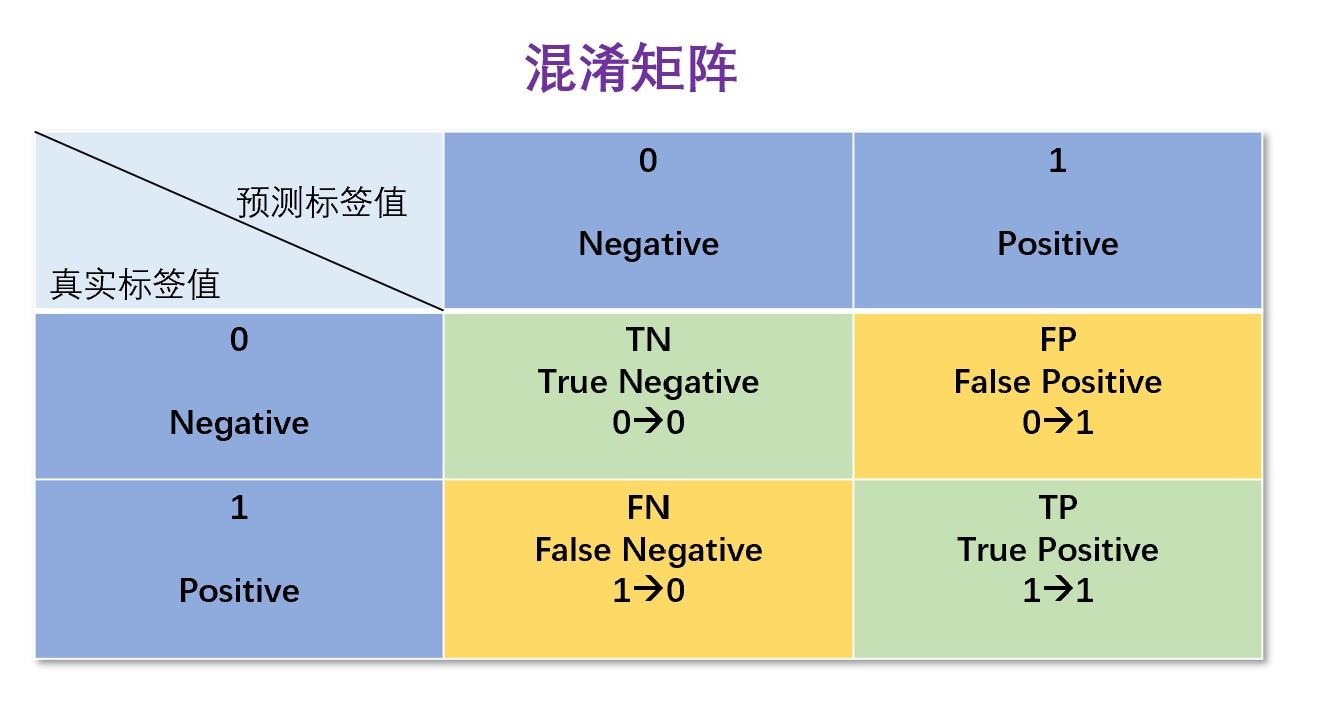• Accuracy（准确率）

衡量预测正确的结果占所有预测结果的比例，混淆矩阵中的TP和TN统计了预测正确的结果数。该指标适合用于类别平衡的数据集上，如果类别不平衡，准确率变得不可靠。比如正例:负例=1:99，那么分类器即使把所有正例都错误预测为负例，也能得到0.99的准确率，这样得到的模型根本无法识别正例。

$Acc=\frac{TN+TP}{TN+TP+FN+FP}$

• Precision（精确率/查准率）

衡量预测为正的结果数有多少实际为正。不要求数据集是平衡的，也适用于不平衡的数据集。

$Precision=\frac{TP}{TP+FP}$

• Recall（召回率/查全率）

衡量实际为正的结果有多少正例被正确预测了。

$Recall=\frac{TP}{TP+FN}$

• P-R曲线

分类模型的原始输出一般是预测为正例的概率，然后将概率值与事先规定好的分类阈值做比较，当概率值大于阈值时，将样本归为正例，否则为负例。得到样本的标签值之后，就可以得到对应该阈值的混淆矩阵，进一步计算该阈值下的P值和R值。以纵坐标为P值，横坐标为R值，将算得的一组P值和R值画到坐标上，就可以得到P-R曲线。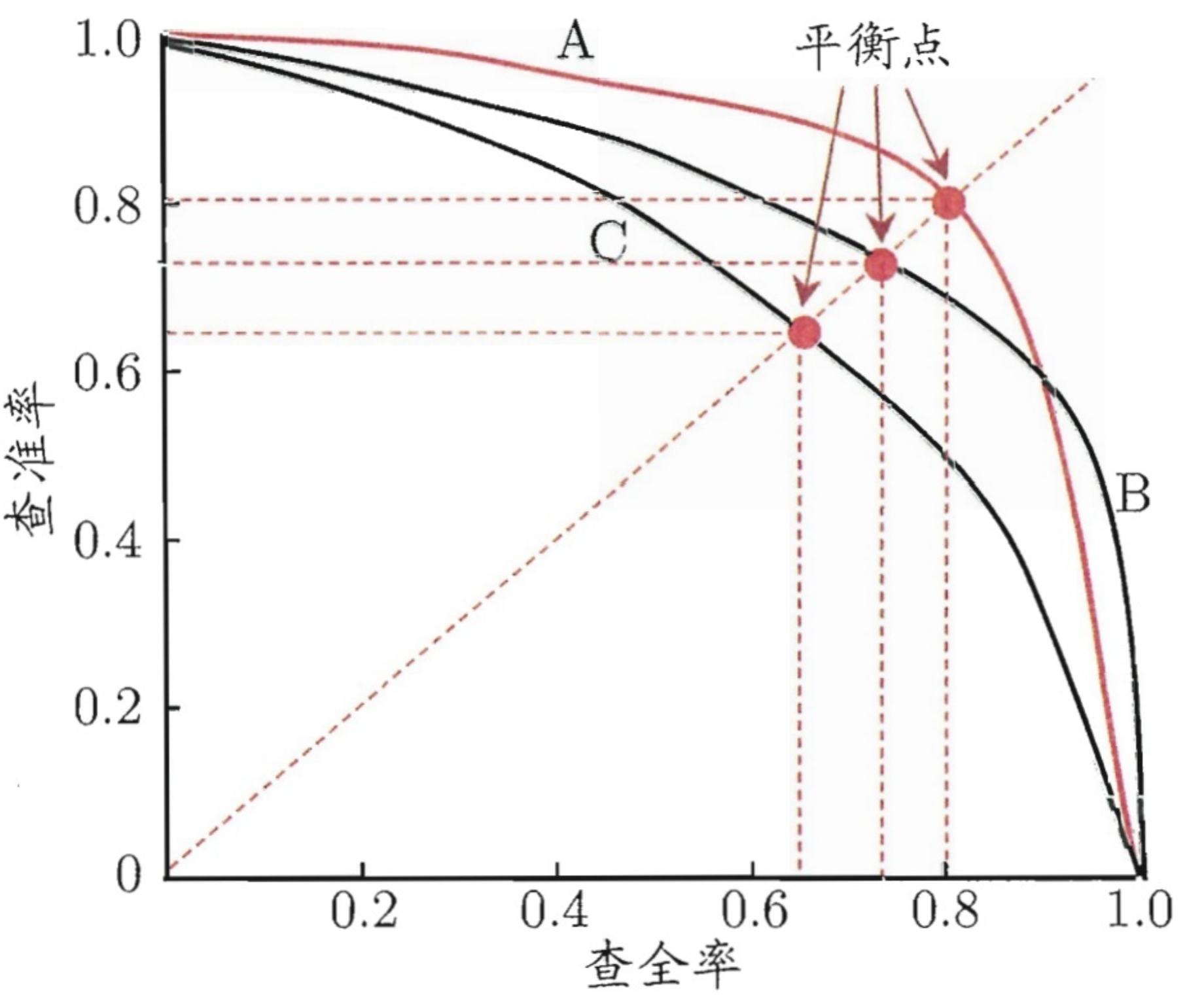如果一个模型的P-R曲线完全包住另一个模型的P-R曲线，则前者的性能优于后者（如A>C，B>C)；

如果两者曲线有交叉(A和B），则使用以下三种比较方法：

• 比较曲线下的面积(即AP，Average Precision，AP常被用作推荐或排序任务的评价指标)
• 平衡点（P=R时的取值），如图，A的平衡点高于B的平衡点，则A优于B
• F1值
• F1 Score（调和平均）

当问题只关注Precision值或者只关注Recall值时，使用Precision或者Recall来评价模型就可以。但是当需要同时关注Precision值和Recall值时，则需要综合这两者的取值。一般我们希望Precision和Recall值都越高越好，但是从P-R曲线就可以看出，这两者是矛盾的，往往是一个高一个低，很难让Precision和Recall都很高。F1则调和了Precision和Recall两者的取值，更综合地评价模型的性能，其适合类别不平衡的数据集。

$F_1 = \frac{2*Precision*Recall}{Precision+Recall}$

ROC的横轴是假阳性率（False Positive Rate，FPR），即假正例占所有负例的比例。 $FPR = \frac{FP}{TN+FP}$ 纵轴就是真阳性率（True Positive Rate，TPR），即真正例占所有正例的比例，等价于召回率。 $TPR = \frac{TP}{TP+FN}$ 绘制ROC曲线与绘制P-R曲线是一样的，即取不同的阈值，计算出来一组FPR和TPR，将这些点可视化后得到ROC曲线。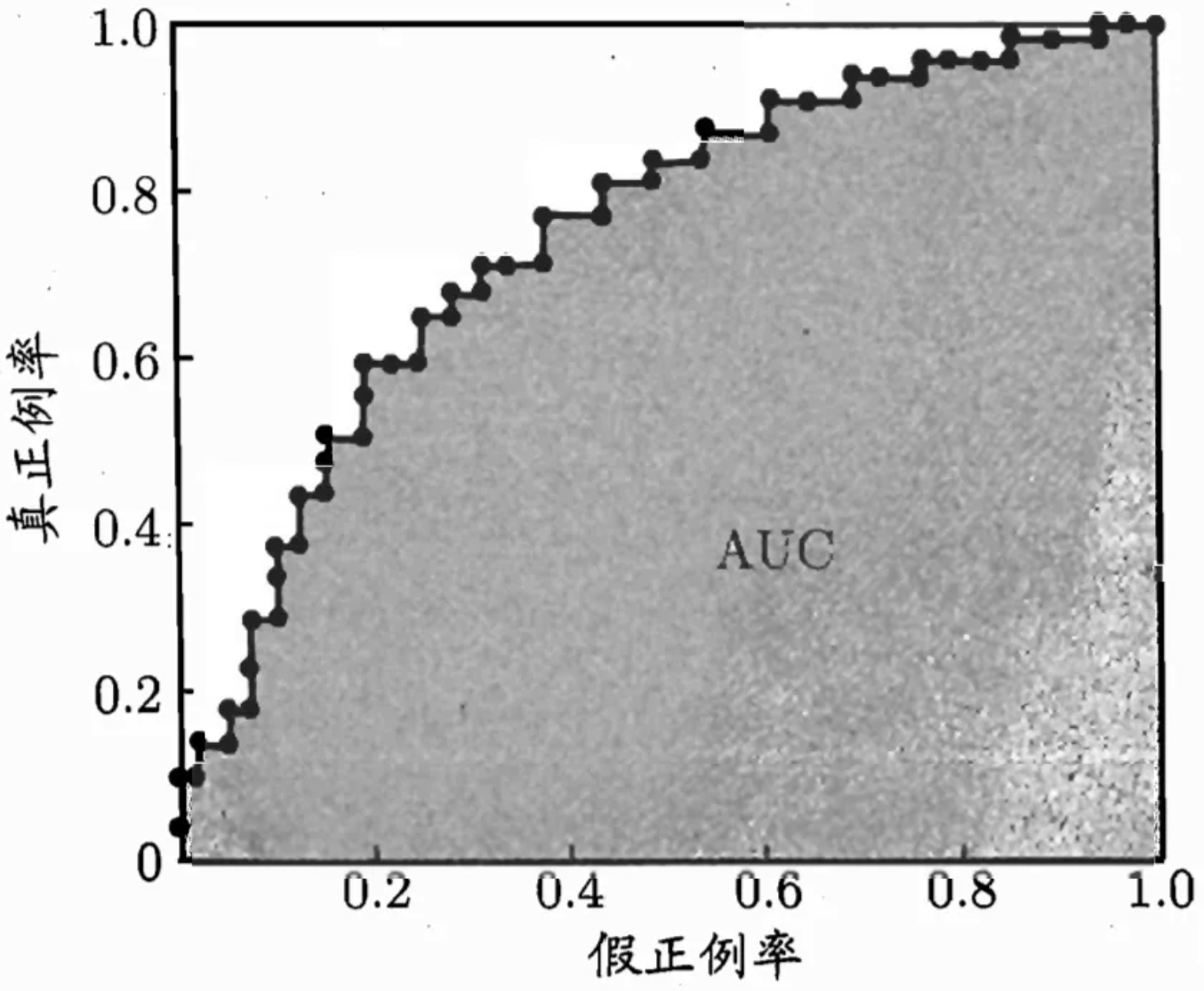可以看出，当ROC曲线越靠近左上角，模型性能越好；

在比较两个模型性能时，如果一个模型的ROC完全包住另一个模型的ROC曲线，则前者的分类性能优于后者；

如果两个模型的ROC曲线有交叉，则计算对比ROC曲线下的面积。

• AUC（Area Under ROC Curve）

ROC曲线下的面积即为AUC。其还有一种定义是：分别随机从测试样本集中抽取一个正负样本，正样本的预测值大于负样本的概率。

假设数据集一共有 $$M$$ 个正样本，$$N$$ 个负样本，预测值也就是 $$M+N$$ 个。我们将所有样本按照预测值进行从小到大排序，并排序编号由1到 $$M+N$$ ，如果出现预测值一样的样本，对这些样本的rank值取平均记为新的rank值。

• 对于正样本概率最大的，假设排序编号为 $$rank_1$$，比它概率小的负样本个数= $$rank_1-M$$
• 对于正样本概率第二大的，假设排序编号为 $$rank_2$$，比它概率小的负样本个数= $$rank_2-(M-1)$$
• 以此类推$$\cdots$$
• 对于正样本概率最小的，假设排序编号为 $$rank_M$$，比它概率小的负样本个数= $$rank_M-1$$

所以，AUC的计算公式如下： $AUC=\frac{\sum_{i\in 正样本}rank(i)-\frac{M(M+1)}{2}}{M\times N}$

有一堆样本，其中2000个负样本，8000个正样本，其中负样本的预测值为均匀分布U(0.4, 0.6)，正样本的预测值为均匀分布U(0.5, 0.7)，求AUC。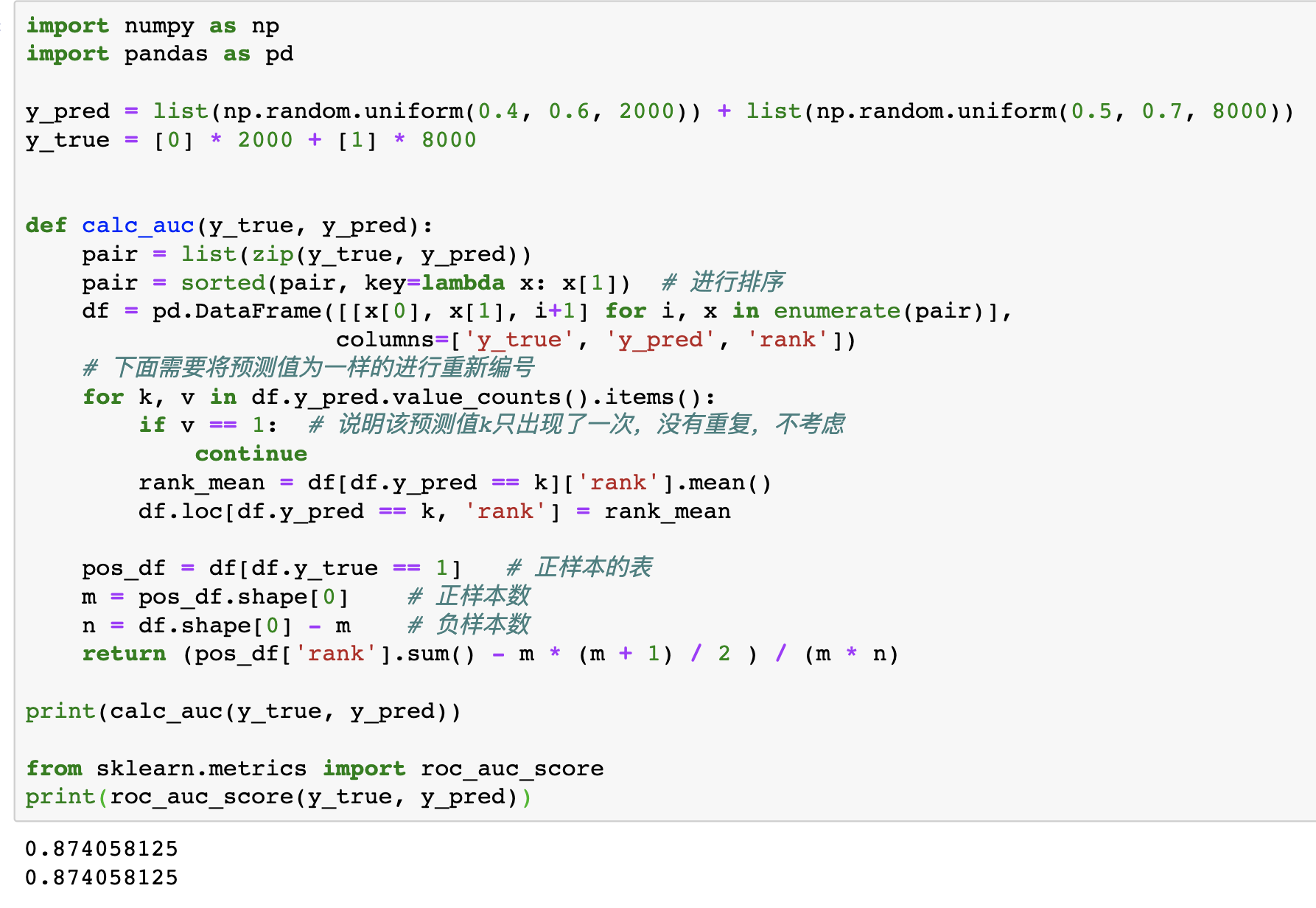##### 1.20.3 AUC的优缺点

• AUC衡量的是一种排序能力，因此特别适合排序类业务；
• AUC对正负样本均衡并不敏感，在样本不均衡的情况下，也可以做出合理的评估；
• 其他指标比如Precision，Recall，F1，根据区分正负样本阈值的变化会有不同的结果，而AUC不需要手动设定阈值，是一种整体上的衡量方法；

• 忽略了预测的概率值和模型的拟合程度；
• AUC反应了太过笼统的信息，无法反应召回率、精确率等在实际业务中经常关心的指标；
• 它没有给出模型误差的空间分布信息，AUC只关注正负样本之间的排序，并不关心正样本内部，或者负样本内部的排序，这样我们也无法衡量样本对于正负样本的好坏程度的刻画能力；
##### 1.20.4 P-R曲线和ROC曲线的区别
• 当测试集中的正负样本的分布变化的时候，ROC曲线能够保持不变；
• 在ROC空间，ROC曲线越向左上方向效果越好，而P-R曲线是越向右上方向效果越好
• ROC曲线由于兼顾正例与负例，所以适用于评估分类器的整体性能(通常是会计算AUC，表示模型的rank性能)，相比而言PR曲线完全聚焦于正例
• 如果有多份数据且存在不同的类别分布，这时候如果只想单纯地比较分类器的性能且剔除类别分布改变的影响，则ROC曲线比较适合，因为类别分布改变可能使得PR曲线发生变化时好时坏，这种时候难以进行模型比较；反之，如果想测试不同类别分布下对分类器的性能的影响，则PR曲线比较适合；
• 如果想要评估在相同的类别分布下正例的预测情况，则宜选PR曲线；类别不平衡问题中，ROC曲线通常会给出一个乐观的效果估计，所以大部分时候还是PR曲线更好。

### 二、推荐系统

#### 2.1 推荐系统特征交叉模型的演变

FFM在此基础上引入field的概念，针对不同的field使用不同的隐向量；

Wide＆Deep是其中一个探索的例子，它以交叉特征作为一个线性模型的输入，与一个DNN模型一起训练，然而，W&D网络的成功取决于正确的交叉特征的选择（仍依赖人工特征工程），这是一个至今还没有明确有效的方法解决的指数问题；

DCN进行进一步探索，将Wide部分替换为由特殊网络结构实现的Cross，自动构造有限高阶的交叉特征，并学习对应权重，告别了繁琐的人工叉乘。这其实应用了残差网络的思想，每一层的输出，都是上一层的输出加上feature crossing f。而f就是在拟合该层输出和上一层输出的残差。残差网络有很多优点，其中一点是处理梯度消失的问题，使网络层数可以“更深”。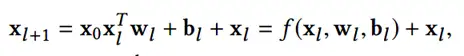DeepFM的模型结构采用Wide&Deep的设计思路，分别使用FMDNN两种结构学习特征的显式低阶隐式高阶交叉信息，再通过输出层将两部分结果结合。另一方面，模型巧妙利用embedding层，使FM部分和DNN部分共享底层特征的emebdding，有利于模型的学习效率。

#### 2.3 推荐模型发展过程

1. 协同过滤CF算法阶段：只需用户物品共现矩阵就可以构建推荐系统，根据相似度取值对象可分为itemCF和userCF两类，优势是简单易实现。CF的问题是泛化能力弱，无法应对稀疏矩阵，而矩阵分解作为协同过滤的进化版，克服了CF的缺点。
2. 逻辑回归LR阶段：综合利用用户、物品、上下文等多种不同的特征，假设用户是否点击广告服从伯努利分布，将推荐问题转化为点击率预估(CTR)问题，预测正样本概率对物品进行排序。其数学形式是各个特征的加权和经过sigmoid函数，得到用户点击物品的概率。LR的优势是可解释性强、易于并行化、模型简单、训练开销小。其局限性在于表达能力不强，需要大量具有业务背景知识的人工特征筛选与交叉。
3. 因子分解机FM阶段：为每个特征学习一个隐向量，在特征交叉时，使用两个特征隐向量的内积作为交叉特征的权重。虽然FM相比POLY2的完全交叉+单一权重记忆能力略弱，但解决了特征交叉过程中交叉特征对应的数据过于稀疏无法充分学习权重的问题。FFM引入特征域进一步增强了模型的表达能力，做特征交叉时，每个特征选择与对方域对应的隐向量的内积作为交叉特征的权重，但FFM的计算复杂度也由kn上升到kn*n。
4. 组合模型阶段：这一阶段主要是为了进一步提高特征交叉的维度，同时融合多个模型的优点。GBDT+LR是组合模型的代表方案，GBDT自动进行特征筛选和组合得到新的离散特征向量输入LR模型。GBDT+LR的组合方式开启了特征工程模型化的趋势，真正实现端到端训练。

（1）特征交叉模型：

• AutoRec：将自编码器(AutoEncoder)与协同过滤结合的单隐层神经网络模型，利用协同过滤中的共现矩阵，完成物品/用户向量的自编码，基于自编码的结果得到用户对物品的预估评分，进而排序。AutoRec模型结构和word2vec结构一致，相对简单，但优化目标和训练方法有所不同，AutoRec表达能力有限。
• DeepFM：2017年由哈工大&华为提出，使用FM替换Wide&Deep的Wide部分，加强浅层网络组合特征的能力。DeepFM的改进目的和Deep&Cross的目的是一致的，只是采用的手段不同。

（2）Attention机制推荐系统的结合：

• AFM：AFM既是FM系列模型的延续演化，也是Attention机制与推荐系统的结合发展。
• DIN：阿里巴巴根据其典型的电商广告推荐场景，于2017年提出DNN结合Attention机制的DIN(Deep Interest Network)模型。利用候选商品和用户历史交互商品之间的相关性得出注意力权重，以此根据用户历史交互商品计算出用户的加权和Embedding。

（3）强化学习与推荐系统的融合：

• DRN：强化学习相比传统深度模型的优势在于强化学习模型能够进行“在线学习”，不断利用新学到的知识更新自己，及时调整和反馈

#### 2.4 Embedding技术在推荐系统中的应用

• word2Vec：w2v是生成对“词”的向量表达的模型。对于由单词组成句子，句子组成文档的语料库，w2v假设每个词都跟其相邻词组成的上下文相关, 或决定上下文(Skip-gram)或由上下文决定(CBOW)。对任意一个句子，目标词前后各选c个词作为上下文，在句子中滑动此长度2c+1的滑动窗口，每移动一次就是一个训练样本。
• Item2Vec：2016年微软提出的Item2Vec与Word2vec基本类似，只是把背景从nlp推广到推荐领域。Item2Vec把用户的浏览、购买等行为交互的物品列表作为”句子”。Item2Vec与Word2Vec唯一的不同在于，Item2Vec中没有时间窗口一说，即用户交互的物品序列中任意两个物品都相关，因此目标函数也是最大化序列中两两物品的对数概率之和。
• DeepWalk：2014年被提出，主要思路是在物品组成的图结构上随机游走，产生大量物品序列，然后基于这些物品序列进行Word2Vec训练得到物品的Embedding。
• Node2Vec：2016年斯坦福提出图数据结构的两种性质：网络的同质性(homophily)，距离相近的节点的Embedding应尽量相似；网络的结构性(structural equivalence)，结构上相似的节点的Embedding应尽量相似。这两种性质可以认为是一阶二阶相似度的扩展，在推荐系统中的解释是，同质性相同的物品很可能是同品类、同属性或经常被一同购买的物品，而结构性相同则是各品类中的爆款、最佳凑单物品。Node2Vec通过调整随机游走权重控制节点间跳转概率进而决定节点Embedding结果倾向于同质性还是结构性。

#### 2.5 离线和线上AUC不一致问题

• 特征穿越是指训练中使用的某些特征包含了样本发生时刻之后的信息，而这些信息往往和label有一定的相关性，导致离线训练时模型根据这类特征对label拟合得非常好，而在线推理时刻的特征只包含了到当前时刻为止的信息而没有未来的信息，导致相关性消失，模型能力明显下降。这类问题一般可以通过定位离在线特征一致性的问题进行改进。

• 模型更新频率依赖于系统的实时性，实时性越高，模型更新越快。一般发展路径为从天级更新到小时级更新，到分钟级更新，最后是实时更新。更新速度越快，对工程的要求越高。当模型更新较慢时，存在离在线数据分布差异较大的问题，从而导致离在线AUC不一致，当模型更新的实时性越高，离线和在线的数据分布差异越小，由分布差异导致的离在线AUC不一致问题将被缓解。

• 样本差异计算线上AUC时，容易忽略两个模型所使用的样本不一致问题，它们的样本集合，分别来自ab实验中实验组和对照组，基于两组不同的用户，模型分别进行线上推理得到各自的线上样本，由于样本不一致，AUC的涨跌增加了随机性，导致离线和在线的AUC涨跌不一致。而模型离线训练时，基线模型和当前模型用于计算AUC的样本来自相同的样本集合，计算出的AUC具有可比性。

业界往往采用两种方式缓解这个问题：

1. 随机样本的方式，即两个模型分别处理相同的用户请求，得到各自的推荐结果，随机选择当前或基线模型的推荐结果曝光给用户，再经过日志处理等系列操作，得到各自的样本；
1. 模型融合的方式，即线上推理时，对两个模型的预估分数进行线性组合，再得到推荐结果。当前模型的权重可以根据流量来调整，随着流量的增大权重可以逐渐增大。

这两种方式本质上都是在削弱基线模型的样本主导优势，因为离线训练时绝大多数样本都是基线模型生成的，当前模型生成的样本只有实验流量这部分，占比很小，这会导致当前模型拟合的是基线模型的样本而非自己产生的样本，而基线模型拟合的就是自己产生的样本，所以在评估时，基线模型有天然的优势。

### 四、概率题

#### 4.1 用rand7构造rand10

Q：已知有个rand7()的函数，其返回1到7随机自然数，让利用这个rand7()构造rand10()随机1~10。

A：要保证rand10()在[1, 10]内均匀分布，可以构造一个1~10*n的均匀分布的整数区间（n为任何正整数）。假设 $$x$$ 是这个[1, 10*n]区间上的一个随机整数，那么 (x-1)%10+1 就是均匀分布在区间 [1, 10] 上的整数。

#### 4.2 山羊汽车问题

Q：参赛者会看见三扇关闭了的门，其中一扇的后面有一辆汽车，选中后面有车的那扇门可赢得该汽车，另外两扇门后面则各藏有一只山羊。当参赛者选定了一扇门，但未去开启它的时候，节目主持人开启剩下两扇门的其中一扇，露出其中一只山羊。主持人其后会问参赛者要不要换另一扇仍然关上的门。问题是：换另一扇门会否增加参赛者赢得汽车的机率？

A：如果严格按照上述的条件，即主持人清楚地知道，自己打开的那扇门后是羊，那么答案是会。不换门的话，赢得汽车的几率是1/3，换门的话，赢得汽车的几率是2/3

#### 4.3 三段组三角形

Q：一根木棍截成三段，形成三角形的概率是多少？

A：1/4

1. 首先，三边长度都大于0，有如下推导公式，易知满足条件的区域面积为 $$\frac{1}{2}*L*L$$

$\left\{ \begin{array}{ccl} x & > & 0 \\ y & > & 0 \\ L-x-y & > & 0 \end{array} \right.$

1. 其次，要构成三角形，必须满足任意两边之和大于第三边，有如下公式 $\left\{ \begin{array}{ccl} x+y & > & L-x-y \\ x+(L-x-y) & > & y \\ y+(L-x-y) & > & x \end{array} \right.$ 画图可知，满足条件的可行域占1/4.

#### 4.4 涂球期望时间

Q：一个木桶里面有M个白球，每分钟从桶中随机取出一个球涂成红色（无论白或红都涂红）再放回，问将桶中球全部涂红的期望时间是多少？

A：$$\frac{M}{1}+\frac{M}{2}+\cdots+\frac{M}{M}$$

$$E(i)$$ 表示木桶里面有 $$i$$ 个红球时，还需要抽取多少次即可将所有球都染成红色。对于当前状态下 $$E(i)$$ 的计算可以分解成两个部分：

1. 抽取到的是红球：需要的期望为 $$i/M*(1+E(i))$$，其中 $$i/M$$ 表示抽取红球的概率，因为抽取到的是红球因此状态没有改变，仍然是 $$E(i)$$

2. 抽取到的是白球：需要的期望为 $$(1-i/M)*(1+E(i+1))$$，其中 $$(1-i/M)$$ 表示抽取白球的概率，因为抽到了白球，被涂成红色后木桶里将会有 $$i+1$$ 个红球，因此状态迁移到 $$E(i+1)$$$E(i)=i/M*(1+E(i))+(1-i/M)*(1+E(i+1))$

#### 4.5 抛骰子期望(1)

Q：抛一个6面的骰子，连续抛直到6为止，问期望的抛的次数是多少？

A：6

1. 1 次抛出 6 的概率为 1/6，那么期望次数为 1*1/6

2. 当前次数抛出的非 6 数字的概率为 5/6，因为没有抛出 6，因此期待抛出 6 还需执行的试验次数仍为 E，需要注意加上本次失效的抛掷，即期望次数为 (1+E)(5/6)$E=1*\frac{1}{6}+(1+E)*(\frac{5}{6})\Rightarrow E=6$

#### 4.6 抛骰子期望(2)

Q：若要使骰子(六个面)的每个数都出现至少一次，那么平均需要掷多少次骰子？即求掷骰子次数的期望

A：$$\frac{6}{1}+\frac{6}{2}+\cdots+\frac{6}{6}$$

#### 4.7 圆内随机选取一点

Q：在半径为1的圆内随机等概率采样一个点

A：从 [0, 2$$\pi$$) 区间内随机选取一个角度，再在这个方向的半径上随机选取一个点。但半径上的点不能均匀选取，选取点的概率要和离圆心的距离成正比，这样才能保证随机点在圆内是均匀分布的。

#### 4.8 平行四边形内随机选取一点

Q：在平行四边形ABCD内随机选取一点

A：两条边AB和AC上各选一点E和F，然后构造平行四边形AEFG，G即为随机选取的在平行四边形之内的点。

#### 4.9 随机发生器期望

Q：已知一随机发生器，产生0的概率是p，产生1的概率是1-p，现在要你构造一个发生器，使得它产生0和1的概率均为1/2。

A：考虑连续产生两个随机数，结果只有四种可能：00、01、10、11，其中产生01和产生10的概率是相等的，均为p*(1-p)，于是可以利用这个概率相等的特性等概率地产生01/10随机数。最终把01映射为0，10映射为1。于是整个方案就是：

#### 4.10 抛硬币吃苹果

Q：两个人轮流抛硬币，规定第一个抛出正面的人可以吃苹果，求先抛的人吃苹果的概率。

A：$$\frac{2}{3}$$

#### 4.11 宝剑升级期望

Q：你有一把宝剑，每使用一个宝石，有50%的概率会成功让宝剑升一级，50%的概率会失败。如果宝剑的级数大于等于5的话，那么失败会使得宝剑降1级。如果宝剑的级数小于5的话，失败没有效果。问题是：期望用多少个宝石可以让一把1级的宝剑升到9级。

A：36次

$$a[i]$$ 表示从第 $$i-1$$ 级升到第 $$i$$ 级期望使用的宝石数量

• $$i \le 5$$ 时，因为不会降级，则期望的数量均为2，即 $$a=a=a=a=2$$

• $$i > 5$$ 时，因为会降级，成功时一个宝石就够了，不成功时需要倒退一级，需要先使用 $$a[i-1]$$ 个宝石回到 $$i-1$$ 级，再使用 $$a[i]$$ 个宝石升到第 $$i$$ 级，即 $a[i] = \frac{1}{2} + \frac{1}{2}(1+a[i-1]+a[i])$ 可知，$$a=4, a=6, a=8, a=10$$.

#### 4.12 扑克牌三等份

Q：一副扑克牌，分成三堆，求大小王出现在同一份的概率。

A：不妨记三份分别为A、B、C。大小王之一肯定在某一份中，不妨假定在A份中，概率为1/3。然后A份只有17张牌可能含有另一张王，而B份、C份则各有18张牌可能含有另一张王，因此A份中含有另一张王的概率是17/(17+18+18)=17/53。也因此可知，A份中同时含有大小王的概率为1/3 * 17/53。 题目问的是出现在同一份中的概率，因此由全概率公式可知所求概率为3(1/3 17/53)=17/53。

### 五、反问

• 小组大概是做什么的，业务方向、具体用到的技术栈和目前遇到的挑战和瓶颈
• 小组成立多久、规模多少人、有哪些base、在公司定位以及资源倾斜、未来几年发展规划
• 怎样培养新人，新人过去大概的上手流程是怎样的，会有多长的适应期
• 小组内的工作强度怎样，每天大概几点上班和几点下班，周末加班吗
• 针对刚刚的面试表现，您觉得我还有哪些方面要再加强一下
• 我最近比较有空，如果您觉得我还ok的话，可以尽快帮我排一下后面的流程微信支付宝